• 累计撰写 67 篇文章
• 累计创建 20 个标签
• 累计收到 37 条评论

### 目 录CONTENT# 链表反转2023-07-25 / 0 评论 / 0 点赞 / 450 阅读 / 1,780 字

# 一、定义链表

package linkedlist;

// 反转链表的五种方式
private final Node head = new Node(null, null);

// 快速填充链表
public void fill() {
Node n5 = new Node(5, null);
Node n4 = new Node(4, n5);
Node n3 = new Node(3, n4);
Node n2 = new Node(2, n3);
}

// 打印链表
public void display() {
while (p != null) {
System.out.print(p.value + " -> ");
p = p.next;
}
}

private static class Node {
private final Object value;
private Node next;

public Node(Object value, Node next) {
this.value = value;
this.next = next;
}
}

public static void main(String[] args) {
}
}


# 二、反转链表

## 2.1 方式一

public ReverseLinkedList reverse1() {
// 创建新的链表
// 遍历当前链表
while (p != null) {
// 首插法
p = p.next;
}
return newList;
}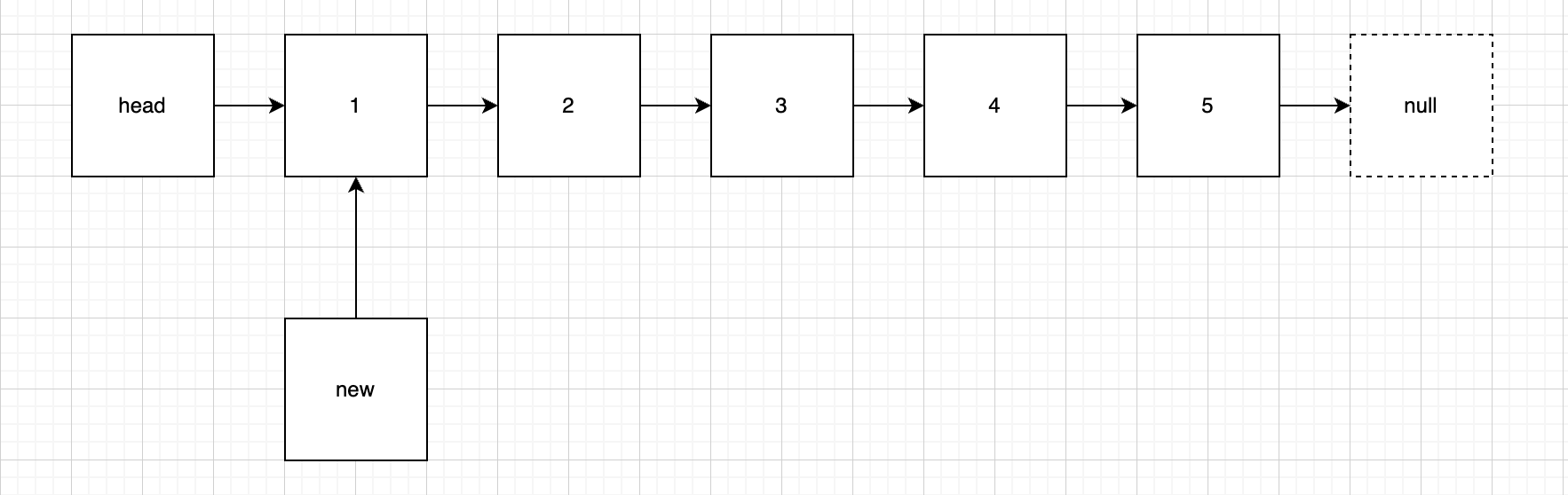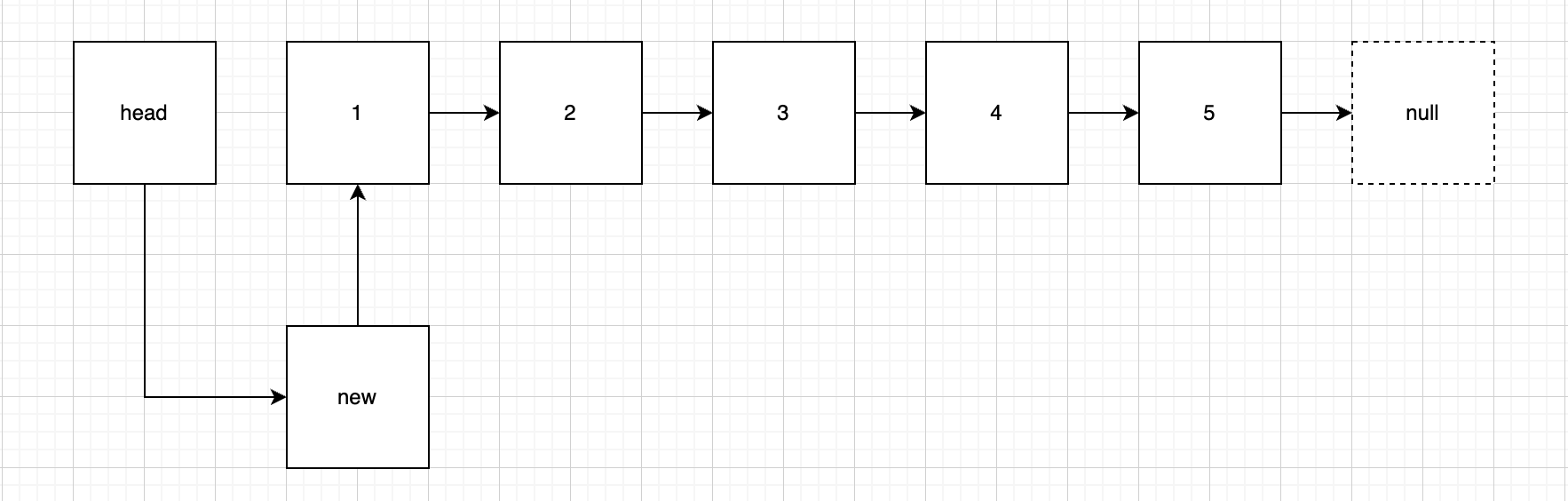## 2.2 方式二

public void addFirst(Node node) {
}


public Node removeFirst() {
// 保存第一个元素
// 判空
if (p != null) {
// 移除第一个元素
}
return p;
}


public ReverseLinkedList reverse2() {
// 依次移除链表元素
Node node = removeFirst();
while (node != null) {
node = removeFirst();
}

return newList;
}


## 2.3 方式三

public void test() {
}

private Node recursion(Node node) {
if (node == null || node.next == null) {
return node; // 最后一个节点
}
return recursion(node.next);
}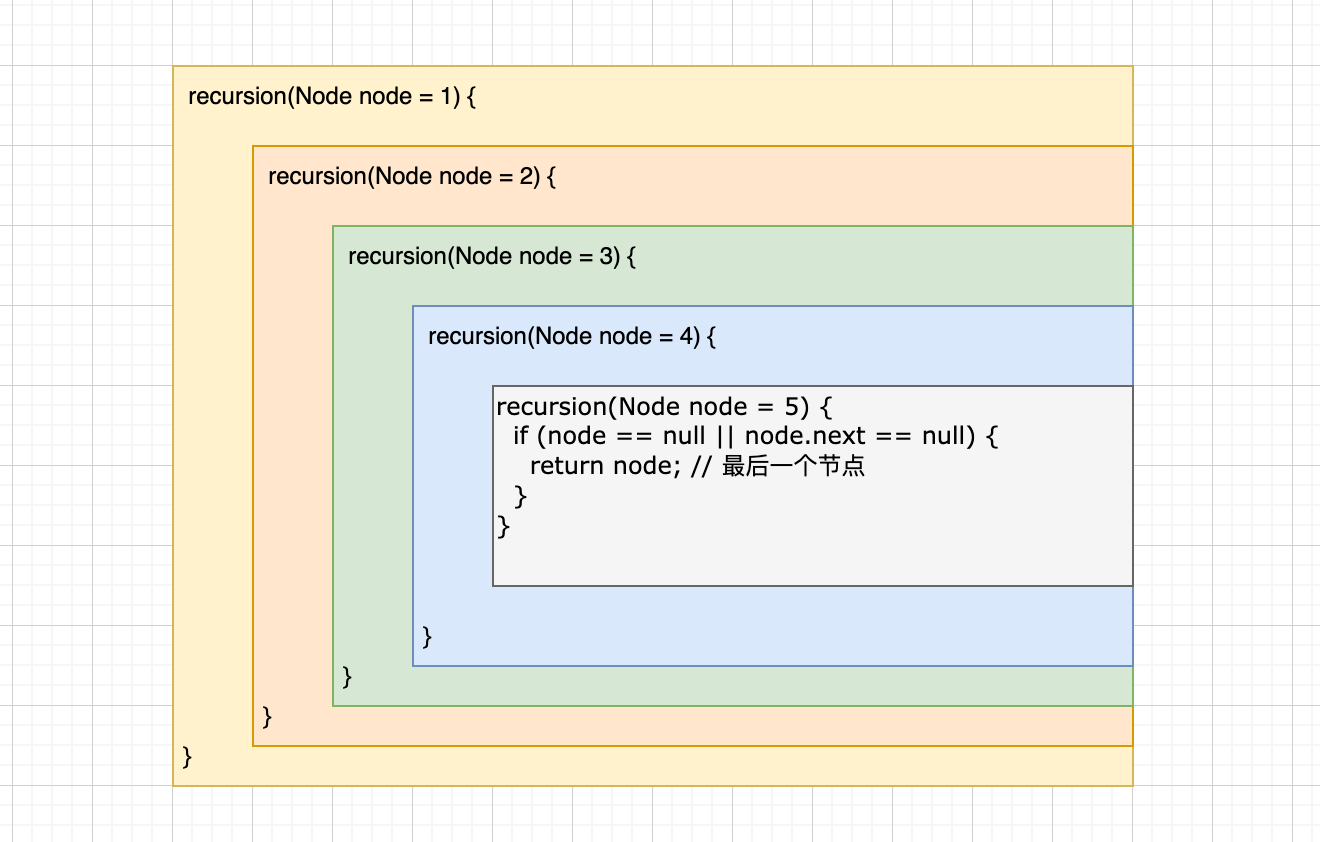private Node recursion(Node node) {
if (node == null || node.next == null) {
return node; // 最后一个节点
}
Node recursion = recursion(node.next);
node.next.next = node;
node.next = null;
return recursion;
}


public void reverse3() {
}


## 2.4 方法四

step-1: 定义三个指针，n1 始终指向链表第一个节点，o1 初始状态指向链表第一个节点，o2 始终指向 o1 的下一个节点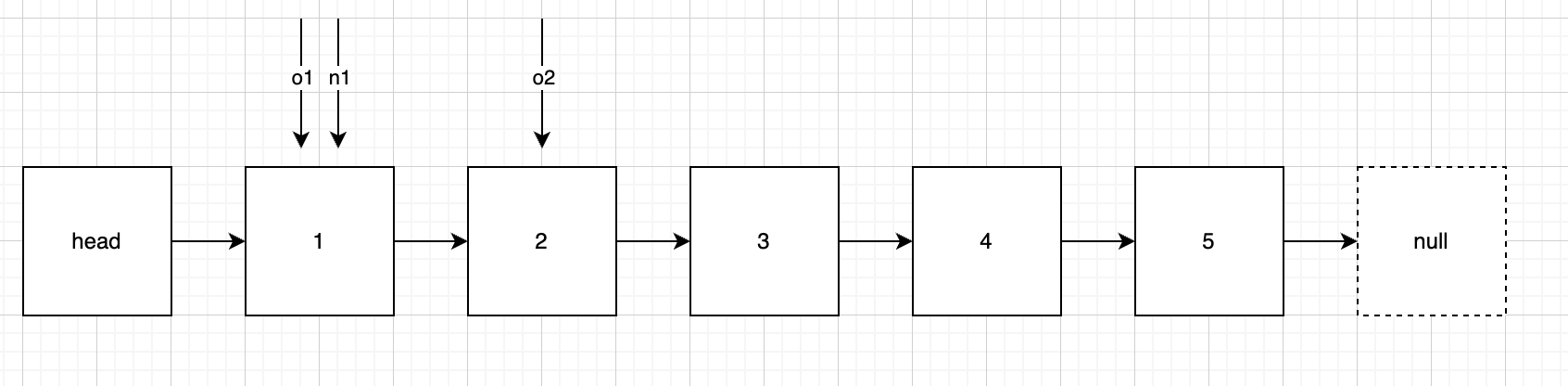step-2: 断开 o2 指向的节点，即o1.next = o2.next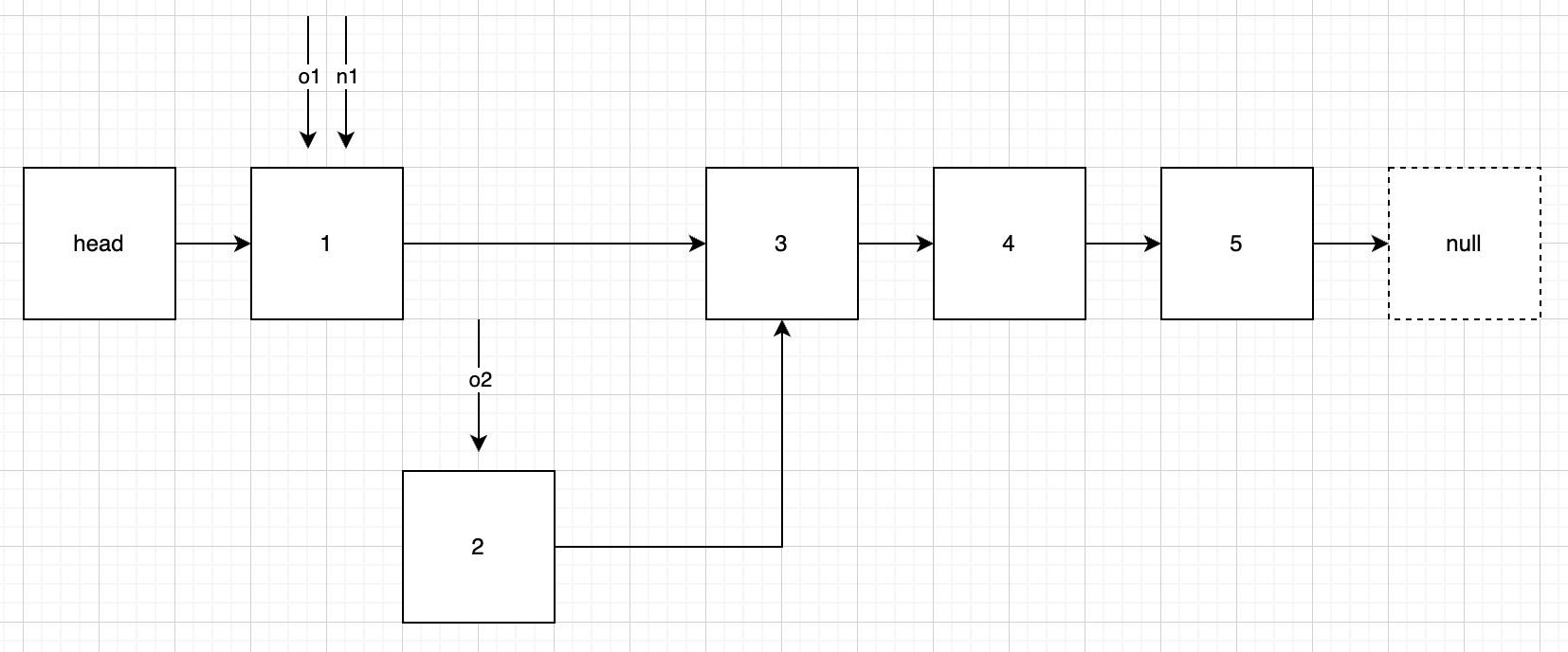step-3: 将 o2 链入到链表头部，即o2.next = n1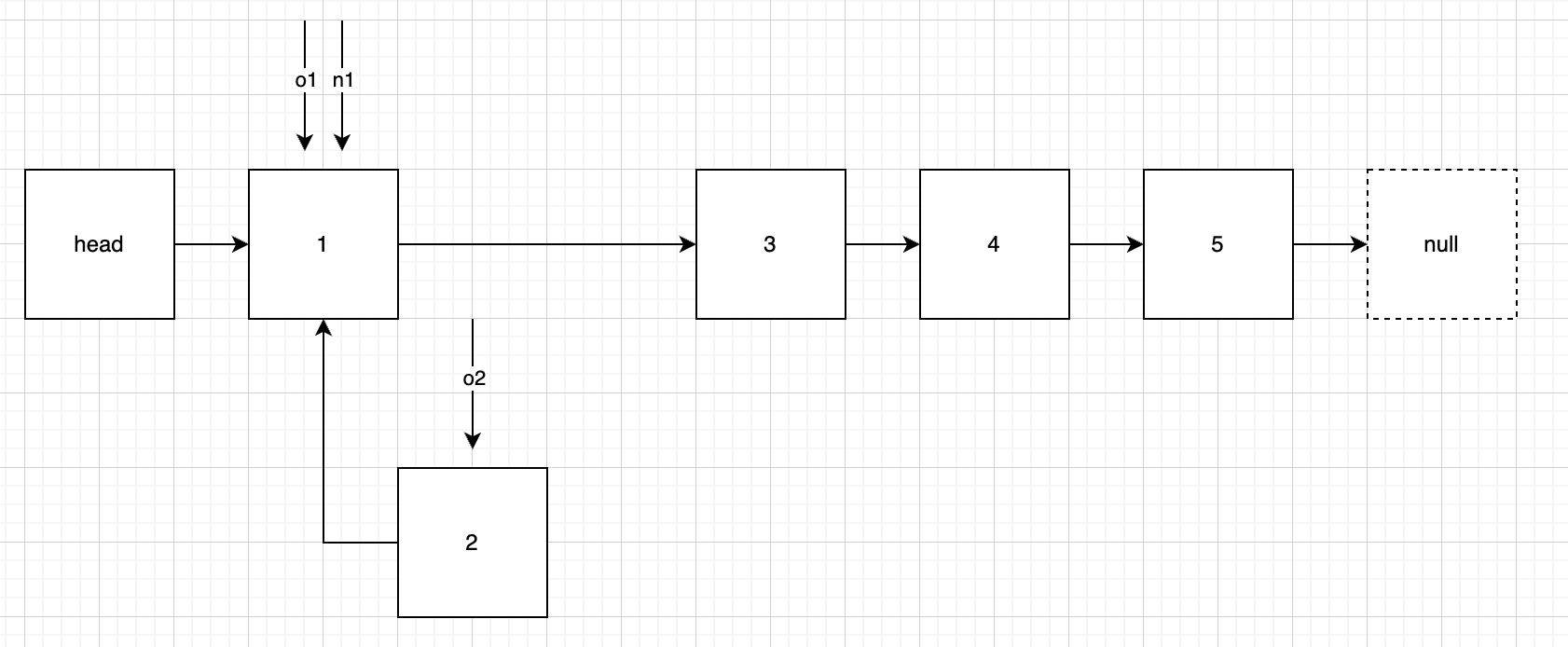step-4: 此时已完成1、2节点的反转，下面需要让 n1 和 o2 回归原本语义，即 n1 始终指向链表头部，那么o1 = o2；o2 始终指向 o1 的下一个节点，那么o2 = o1.next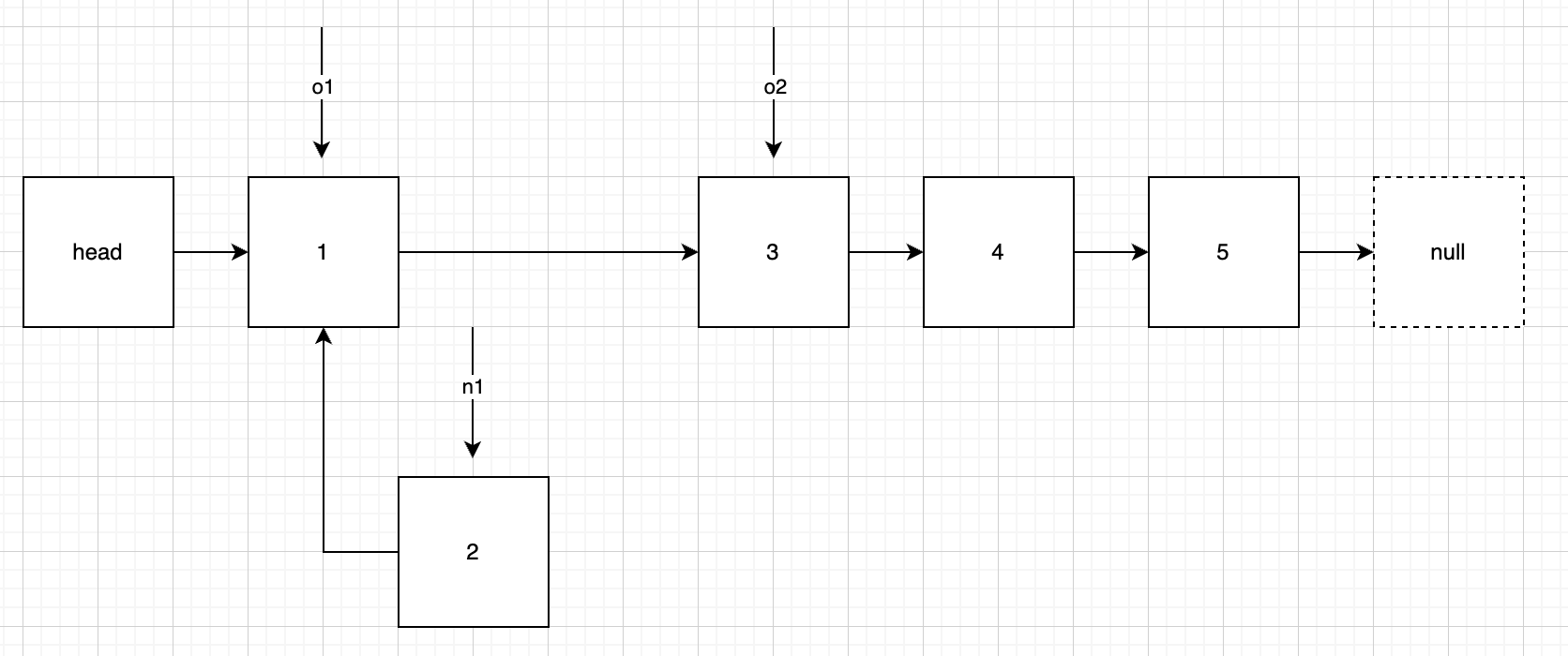step-5: 重复 step-2 直到 o2 = null

public void reverse4() {
// step-1: 定义三个指针，n1 始终指向链表第一个节点，o1 初始状态指向链表第一个节点，o2 始终指向 o1 的下一个节点
if (o1 == null) {
// 空链表
return;
}
Node o2 = o1.next;
// step-5: 重复 step-2 直到 o2 = null
while (o2 != null) {
// step-2: 断开 o2 指向的节点
o1.next = o2.next;
// step-3: 将 o2 链入到链表头部
o2.next = n1;
// step-4: 此时已完成1、2节点的反转，下面需要让 n1 和 o2 回归原本语义
n1 = o2;
o2 = o1.next;
}
}


## 2.5 方法五

step-1: 定义两个指针，o1 始终指向老链表头部，n1 始终指向新链表头部，初始为 null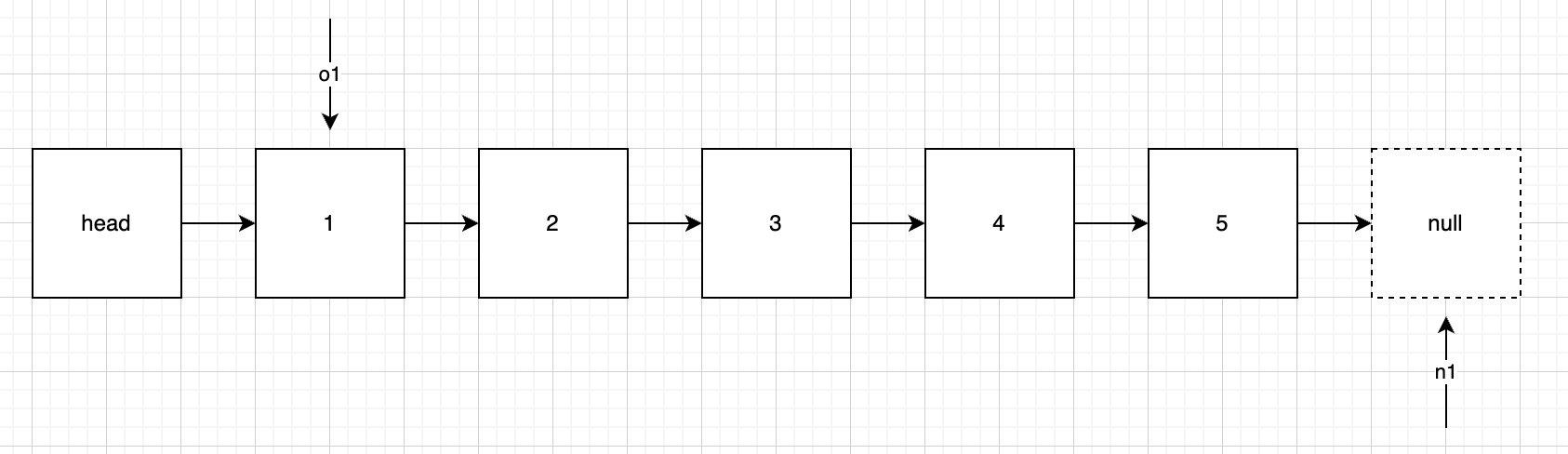step-2: 先定义一个辅助节点 o2 指向 o1 的下一个节点，然后将 o1 指向的节点链入 n1 新链表的头部。o2 的作用是让 o1 能回归原本的语言（当然用 head 回去也是一样的，只不过要平凡动 head）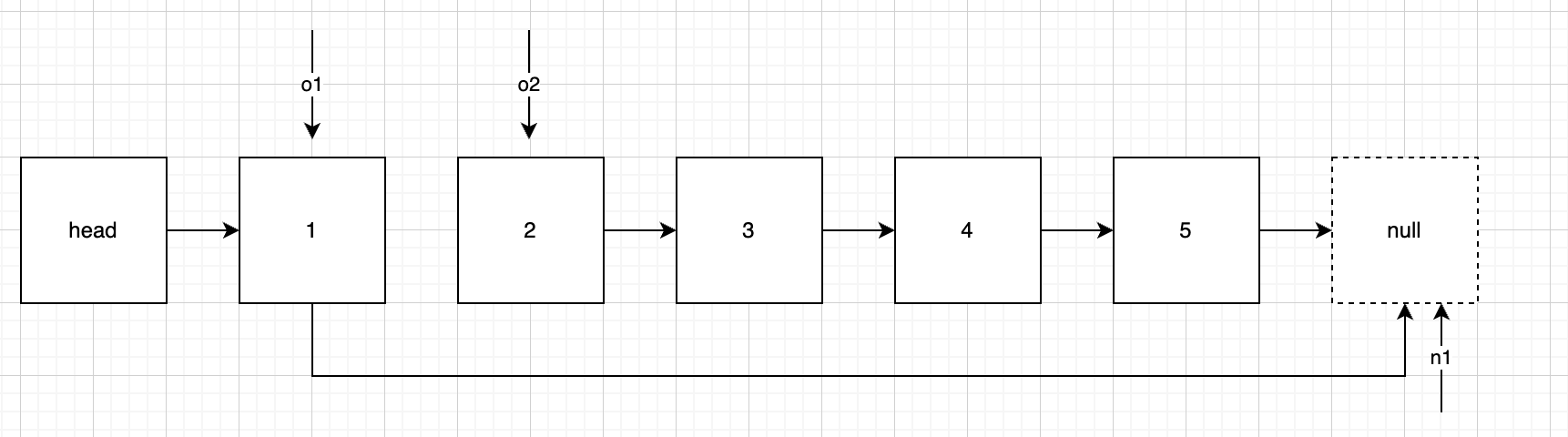step-3: n1、o1 归位，即 n1 始终指向新链表头部，o1 始终指向老链表头部，这里 o1 需要借助 o2 才能归为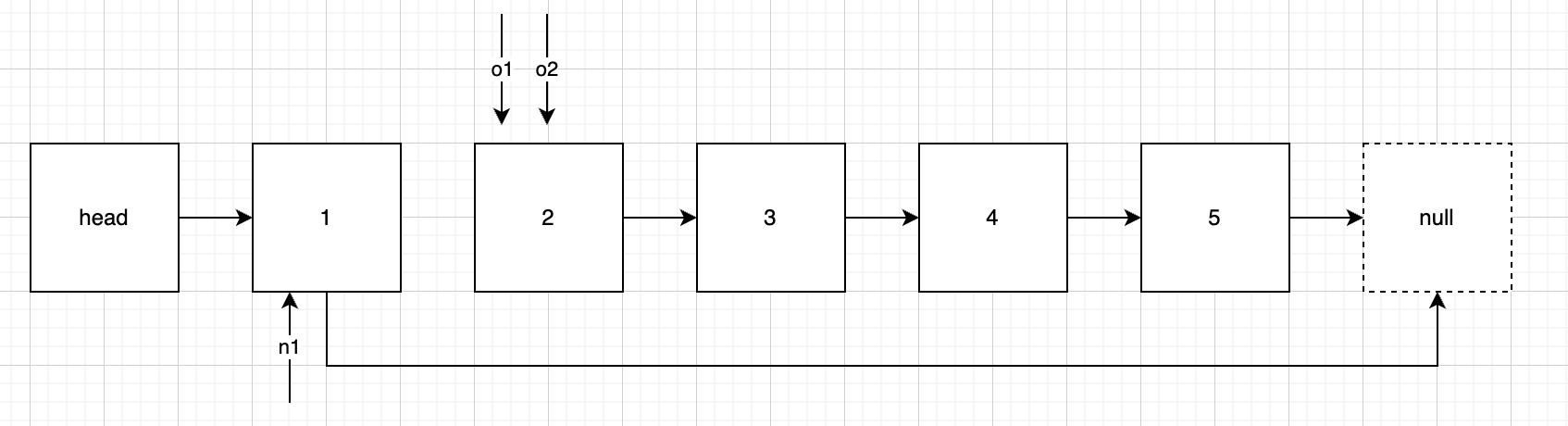step-4: 如果 o1 不指向 null 则回到 step-2，否则结束

public void reverse5() {
// step-1: 定义两个指针，o1 始终指向老链表头部，n1 始终指向新链表头部，初始为 null
Node n1 = null;
// step-4: 如果 o1 不指向 null 则回到 step-2，否则结束
while (o1 != null) {
// step-2: 先定义一个辅助节点 o2 指向 o1 的下一个节点，然后将 o1 指向的节点链入 n1 新链表的头部
Node o2 = o1.next;
o1.next = n1;
// step-3: n1、o1 归位，即 n1 始终指向新链表头部，o1 始终指向老链表头部
n1 = o1;
o1 = o2;
}